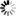Welcome to Central Library, SUST
Normal view

# Configurations from a Graphical Viewpoint [electronic resource] / by Tomaž Pisanski, Brigitte Servatius.

Material type:TextPublisher: Boston : Birkhäuser Boston : Imprint: Birkhäuser, 2013Description: XIII, 279 p. 274 illus., 45 illus. in color. online resourceContent type:
• text
Media type:
• computer
Carrier type:
• online resource
ISBN:
• 9780817683641
Additional physical formats: Printed edition:: No titleDDC classification:
• 511.5 23
LOC classification:
• QA166-166.247
Online resources:
Contents:
Preface -- Introduction -- Graphs -- Groups, Actions, and Symmetry -- Maps -- Combinatorial Configurations -- Geometric Configurations -- Index -- Bibliography.
Summary: Configurations can be studied from a graph-theoretical viewpoint via the so-called Levi graphs and lie at the heart of graphs, groups, surfaces, and geometries, all of which are very active areas of mathematical exploration. In this self-contained textbook, algebraic graph theory is used to introduce groups; topological graph theory is used to explore surfaces; and geometric graph theory is implemented to analyze incidence geometries. After a preview of configurations in Chapter 1, a concise introduction to graph theory is presented in Chapter 2, followed by a geometric introduction to groups in Chapter 3. Maps and surfaces are combinatorially treated in Chapter 4. Chapter 5 introduces the concept of incidence structure through vertex colored graphs, and the combinatorial aspects of classical configurations are studied. Geometric aspects, some historical remarks, references, and applications of classical configurations appear in the last chapter. With over two hundred illustrations, challenging exercises at the end of each chapter, a comprehensive bibliography, and a set of open problems, Configurations from a Graphical Viewpoint is well suited for a graduate graph theory course, an advanced undergraduate seminar, or a self-contained reference for mathematicians and researchers.
Tags from this library: No tags from this library for this title.
Star ratingsAverage rating: 0.0 (0 votes)
No physical items for this record

Preface -- Introduction -- Graphs -- Groups, Actions, and Symmetry -- Maps -- Combinatorial Configurations -- Geometric Configurations -- Index -- Bibliography.

Configurations can be studied from a graph-theoretical viewpoint via the so-called Levi graphs and lie at the heart of graphs, groups, surfaces, and geometries, all of which are very active areas of mathematical exploration. In this self-contained textbook, algebraic graph theory is used to introduce groups; topological graph theory is used to explore surfaces; and geometric graph theory is implemented to analyze incidence geometries. After a preview of configurations in Chapter 1, a concise introduction to graph theory is presented in Chapter 2, followed by a geometric introduction to groups in Chapter 3. Maps and surfaces are combinatorially treated in Chapter 4. Chapter 5 introduces the concept of incidence structure through vertex colored graphs, and the combinatorial aspects of classical configurations are studied. Geometric aspects, some historical remarks, references, and applications of classical configurations appear in the last chapter. With over two hundred illustrations, challenging exercises at the end of each chapter, a comprehensive bibliography, and a set of open problems, Configurations from a Graphical Viewpoint is well suited for a graduate graph theory course, an advanced undergraduate seminar, or a self-contained reference for mathematicians and researchers.

There are no comments on this title.